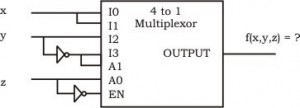# GATE | GATE-CS-2002 | Question 27

• Last Updated : 19 Nov, 2018

Consider the following multiplexor where 10, 11, 12, 13 are four data input lines selected by two address line combinations A1A0 = 00, 01, 10, 11 respectively and f is “the output of the multiplexor. EN is the enable input.The function f(x, y, z) implemented by the above circuit is :
(A) xyz’
(B) xy + z
(C) x + z
(D) None of these

Explanation: F = (A1’A0’I0 + A1’A0I1 + A1A0’I2 + A1A0I3) * EN

F = (XYZ’ +XYZ + Y’ZY + ZY’)Z’
F = (XYZ’ +XYZ + ZY'(Y + 1))Z’
F = (XYZ’ +XYZ + ZY’ * 1)Z’
F = (XY(Z’ + Z) + ZY’)Z’
F = (XY + ZY’)Z’
F = XYZ’ + 0
F = XYZ’

Thus, option (A) is correct.

Please comment below if you find anything wrong in the above post.

Quiz of this Question

My Personal Notes arrow_drop_up